Problem 10:

A particle at the center of a 10 x 1 rectangle undergoes Brownian motion (i.e. 2D random walk with infinitesimal step lengths) till it hits the boundary.  What is the probability that it hits at one of the ends rather than at one of the sides?

Solution:

This is another standard heat equation problem, this time just for the steady state.  The probability is the temperature at the centre in the steady-state solution of the heat equation on the rectangle, where the temperature is fixed at 1 on the ends (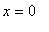and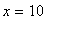) and 0 on the sides (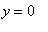and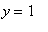).  The solution with temperature 1 atand 0 on the rest of the boundary is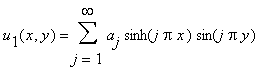where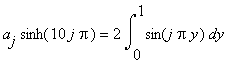:

 > restart; aj:= 2/sinh(10*Pi*j)*int(sin(j*Pi*y),y=0..1) assuming j::integer;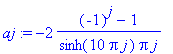> u1:= Sum(aj*sinh(j*Pi*x)*sin(j*Pi*y),j=1..infinity);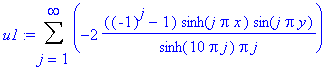By symmetry, the solution with temperature 1 atand 0 on the rest of the boundary is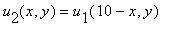.  By superposition, the solution with temperature 1 at both ends is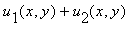.  At the centre, we have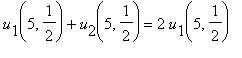.  The series converges rapidly.  Here it is calculated with Digits = 40.

 > Digits:= 40; ans:= 2*evalf(eval(u1,{x=5,y=1/2}));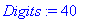This has 39 correct digits.